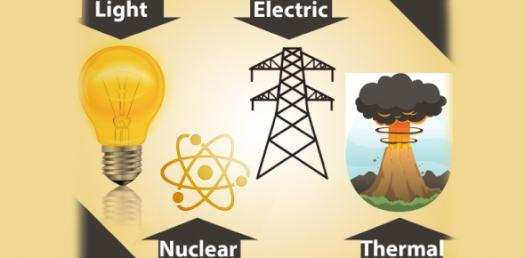# Quiz On Forms Of Energy (Solar, Heat, Sound, And Motion)

Approved & Edited by ProProfs Editorial Team
At ProProfs Quizzes, our dedicated in-house team of experts takes pride in their work. With a sharp eye for detail, they meticulously review each quiz. This ensures that every quiz, taken by over 100 million users, meets our standards of accuracy, clarity, and engagement.
| Written by D_omel
D
D_omel
Community Contributor
Quizzes Created: 12 | Total Attempts: 70,876
Questions: 13 | Attempts: 1,202SettingsComplete the quiz. If you do not pass the quiz, you must retake the quiz.

• 1.

### Food is an example of.....

• A.

Chemical energy.

• B.

• C.

Gravitational energy.

• D.

Electric energy.

• E.

Nuclear energy.

A. Chemical energy.
Explanation
We burn food for energy. Burning is a chemical reaction so food is chemical energy.

Rate this question:

• 2.

### Fuel, like gasoline, is an example of...

• A.

Chemical energy

• B.

Sound energy

• C.

• D.

Nuclear energy

• E.

Electric energy

A. Chemical energy
Explanation
We burn fuel. Burning is a chemical reaction so fuel is chemical energy.

Rate this question:

• 3.

### A rock sitting on the edge of a cliff is an example of...

• A.

• B.

Gravitational energy.

• C.

Nuclear energy.

• D.

Chemical energy.

• E.

Sound energy.

B. Gravitational energy.
Explanation
Anything above the ground is pulled on by gravity so it has gravitational energy.

Rate this question:

• 4.

### A plucked guitar string has...

• A.

Gravitational energy.

• B.

• C.

Sound energy.

• D.

Chemical energy.

• E.

Thermal energy.

C. Sound energy.
Explanation
Anything object that vibrates can produce sound waves so it has sound energy.

Rate this question:

• 5.

### A hot pan has

• A.

Chemical energy.

• B.

Nuclear energy.

• C.

• D.

Electric energy.

• E.

Thermal energy.

E. Thermal energy.
Explanation
Anything that has a temperature has thermal energy.

Rate this question:

• 6.

### Which of the following has thermal energy.

• A.

A refrigerator.

• B.

A hot stove.

• C.

The sun.

• D.

• E.

Explanation
Anything that has a temperature has thermal energy.

Rate this question:

• 7.

### Throwing a baseball is an example of....

• A.

Chemical energy.

• B.

• C.

Nuclear energy.

• D.

Motion energy.

• E.

Gravitational energy.

D. Motion energy.
Explanation
Anything moving has motion energy.

Rate this question:

• 8.

### A stretched rubber band is an example of....

• A.

Gravitational energy.

• B.

Stored mechanical energy.

• C.

• D.

Sound energy.

• E.

Chemical energy.

B. Stored mechanical energy.
Explanation
Anything that is being pulled apart or squeezed together but resists has stored mechanical energy.

Rate this question:

• 9.

### Squeezing a spring is an example of....

• A.

Chemical energy.

• B.

Nuclear energy.

• C.

Thermal energy.

• D.

Stored mechanical energy.

• E.

D. Stored mechanical energy.
Explanation
Anything that is being pulled apart or squeezed together but resists has stored mechanical energy.

Rate this question:

• 10.

### Which of the following are all examples of radiant energy?

• A.

Microwaves, sound waves, light waves, radio waves

• B.

Light waves, fission, sound waves, x-rays

• C.

Light waves, u-v rays, x-rays, microwaves, radiowaves

• D.

• E.

Microwaves, sound waves, earthquake waves

C. Light waves, u-v rays, x-rays, microwaves, radiowaves
Explanation
Any form of light is radiant energy. All the forms of light are as follows: radio waves, microwaves, infrared waves, visible light waves, u-v rays, x-rays, gamma-rays.

Rate this question:

• 11.

### When you turn on a battery powered toy, what energy is moving through the battery and toy?

• A.

Electric energy.

• B.

Gravitational energy.

• C.

Thermal energy.

• D.

• E.

Motion energy.

A. Electric energy.
Explanation
Electricity moves through the toy. Electricity is moving electrons. Moving electrons is electric energy.

Rate this question:

• 12.

### Nuclear energy comes from splitting apart ....

• A.

Two molecules.

• B.

An electron into quarks.

• C.

An atom's nucleus.

C. An atom's nucleus.
Explanation
Nuclear energy comes from the forces that hold together the protons and neutrons in a nucleus. Splitting an atom's nucleus releases nuclear energy.

Rate this question:

• 13.

### How fast molecules move within a matter gives matter...

• A.

Thermal energy.

• B.

• C.

Chemical energy.

• D.

Electric energy.

• E.

Sound energy.Back to top